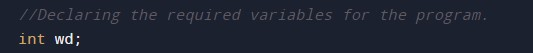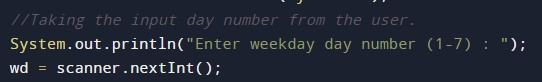# Java Program to Print Name Of Day

In this tutorial you will learn about the Java Program to Print Name Of Day and its application with practical example.

## Java Program to Print Name Of Day

In this tutorial, we will learn to create a Java program that will Print Name Of Day using Java programming.

## Prerequisites

Before starting with this tutorial, we assume that you are the best aware of the following Java programming topics:

• Operators in Java Programming.
• Basic Input and Output function in Java Programming.
• Basic Java programming.
• Conditional Statements in Java programming.
• Arithmetic operations in Java Programming.

## Find the Day Name of a Week:-

In today’s program, we will take the input day in a range between 1 to 7 from the user. Then we will pass that variable to the conditional statements to find the name of days. At last, we will print the resulting name of the day to the user.

With the help of the below program, we can find the name of the day in a week.

## Output:-In the above program, we have first initialized the required variable.• wd = it will hold the integer value for the input.

Taking the input day from the user.Finding the name of the day in a week using conditional statements.Printing the output of the program.In this tutorial we have learn about the Java Program to Print Name Of Day and its application with practical example. I hope you will like this tutorial.